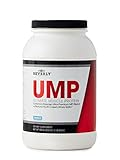ContentsFat Ratio

### Tags

Fat Ratio
Fat Ratio (%)

Below is the degree of change seen after Fat Ratio is higher than average.

Below is the change in Fat Ratio seen after the predictor is higher than average.

Outcomes
of
Below is the change in the listed outcome after is higher than average.
Sort by Effect Size
Sort by Confidence
% Change from Baseline*
*
Change in the listed outcome after is higher than average.
Predictors
of
Below is the change in after the listed predictor is higher than average.
Sort by Effect Size
Sort by Confidence
% Change from Baseline*
*
Change from the average seen after the predictor is higher than average.

### Fat Ratio Info

Property Value
Variable Name Fat Ratio (%)
Aggregation Method MEAN
Analysis Performed At 2020-10-09
Duration of Action 7 days
Kurtosis 1.5493821176958
Mean 25.432071428571 percent
Median 25.432071428571 percent
Number of Aggregate Predictors 58
Number of Aggregate Outcomes 37
Number of Measurements 128
Number of Measurements (including those generated by tagged, joined, or child variables) 128
Public true
Onset Delay 0 seconds
Standard Deviation 1.5626121466754
Unit Percent
User Variables 7
UPC 795186416775
Variable Category Physique
Variable ID 5930060
Variance 4.2796280330714